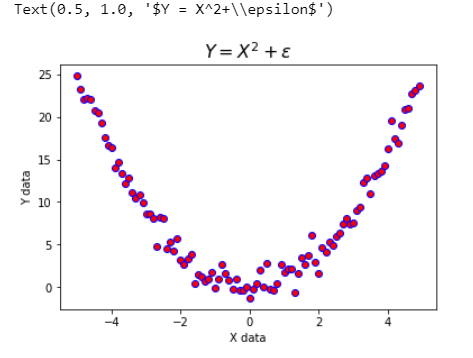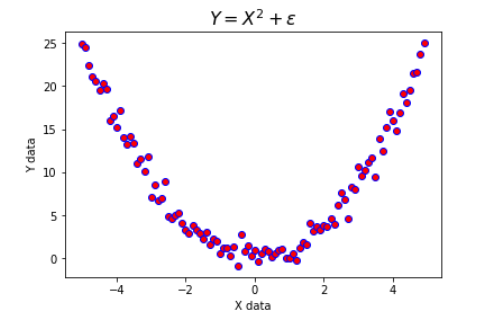Search

# What is Pearson's-r Correlation coefficient In Machine Learning ? - Data Dependency

#### Data Dependency

###### Pearson's-r Correlation coefficientImport Libraries

import numpy as np
import csv
import matplotlib.pyplot as plt
import scipy.stats
import pandas as pd

%matplotlib inline

!pip install wget

import wget



data = pd.read_csv('Auto.csv')

Selecting Record

data.head()Describe Dataset

data.describe()Selecting Two features

miles = data['miles']
weights = data['Weight']
print miles[:10]
print weights[:10]

pearson_r = np.cov(miles, weights)[0, 1] / (miles.std() * weights.std())
print pearson_r

Finding correlation Coefficient of each features

np.corrcoef(miles,weights)
horse = data['Horse power']
np.corrcoef(weights,horse)

Plot

# plotting
fig, ax = plt.subplots(figsize=(7, 5), dpi=300)
ax.scatter(weights,miles, alpha=0.6, edgecolor='none', s=100)
ax.set_xlabel('Car Weight (tons)')
ax.set_ylabel('Miles Per Gallon')

line_coef = np.polyfit(weights, miles, 1)
xx = np.arange(1, 5, 0.1)
yy = line_coef*xx + line_coef

ax.plot(xx, yy, 'r', lw=2)



Output:Practice Exercise

1. Find the Pearson's-r coefficient for two linearly dependent variables. Add some noise and see the effect of varying the noise.

2. Simulate and visualize some data with positive linear correlation

3. Simulate and visualize some data with negative linear correlation.

xx = np.arange(-5, 5, 0.1)
pp = 1.5  # level of noise
yy = xx + np.random.normal(0, pp, size=len(xx))

# visualize the data
fig, ax = plt.subplots()
ax.scatter(xx, yy, c='r', edgecolor='none')
ax.set_xlabel('X data')
ax.set_ylabel('Y data')

line_coef = np.polyfit(xx, yy, 1)
line_xx = np.arange(-5, 5, 0.1)
line_yy = line_coef*line_xx + line_coef

ax.plot(line_xx, line_yy, 'b', lw=2)

print scipy.stats.pearsonr(xx, yy)

OutputPearson's r coefficient is limited to analyze the linear correlation between two variables. It is not capable to show the non-linear dependency. Investigate the Pearson's r coefficient between two variables that are correlated non-linearly.

# generate some data, first for X
xx = np.arange(-5, 5, 0.1)

# assume Y = 2Y + some perturbation
pp = 1.1  # level of noise
yy = xx**2 + np.random.normal(0, pp, size=len(xx))

# visualize the data
fig, ax = plt.subplots()
ax.scatter(xx, yy, c='r', edgecolor='b')
ax.set_xlabel('X data')
ax.set_ylabel('Y data')
ax.set_title('$Y = X^2+\epsilon$', size=16)

Output:# generate some data, first for X
xx = np.arange(-5, 5, 0.1)

# assume Y = 2Y + some perturbation
pp = 1.1  # level of noise
yy = xx**2 + np.random.normal(0, pp, size=len(xx))

# visualize the data
fig, ax = plt.subplots()
ax.scatter(xx, yy, c='r', edgecolor='b')
ax.set_xlabel('X data')
ax.set_ylabel('Y data')
ax.set_title('$Y = X^2+\epsilon$', size=16)



Output:The Pearson's-r correlation is near zero which means there is no linear correlation. But how about non-linear correlation? Isn't y=x2?

np.corrcoef(xx,yy)

Output:

array([[ 1.        , -0.04489687],
[-0.04489687,  1.        ]])